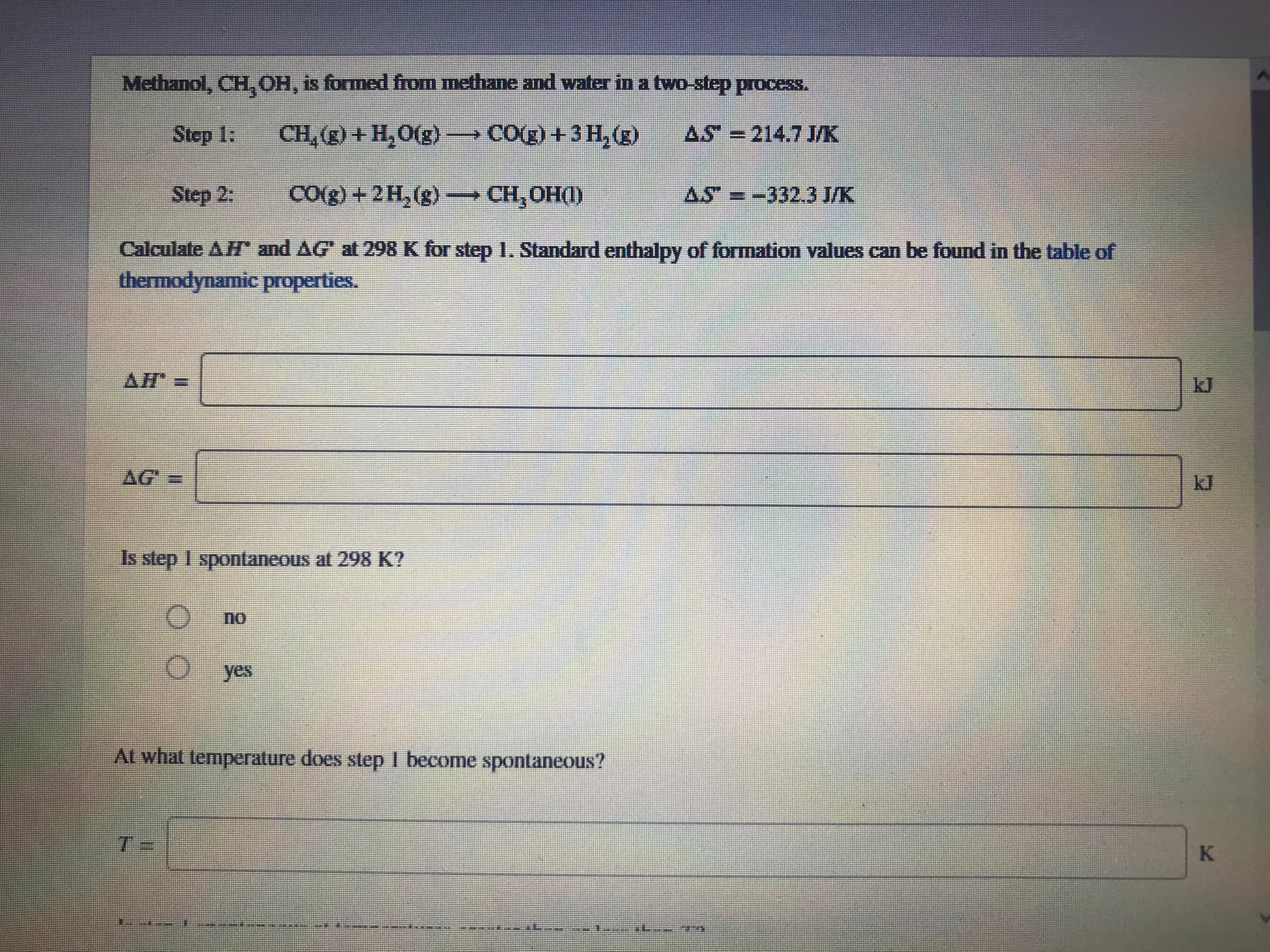# Methanol , CH3OH, is formed from methane and water in a two-step process. Step 1: CH4(g) + H2O(g) → CO(g) + 3 H2(g) ΔS° = 214.7 J/K Step 2: CO(g) + 2 H2(g) → CH3OH(l) ΔS° = -332.3 J/K Calculate ΔH° and ΔG° at 298 K for step 1. Standard enthalpy of formation values can be found in the table of thermodynamic properties.